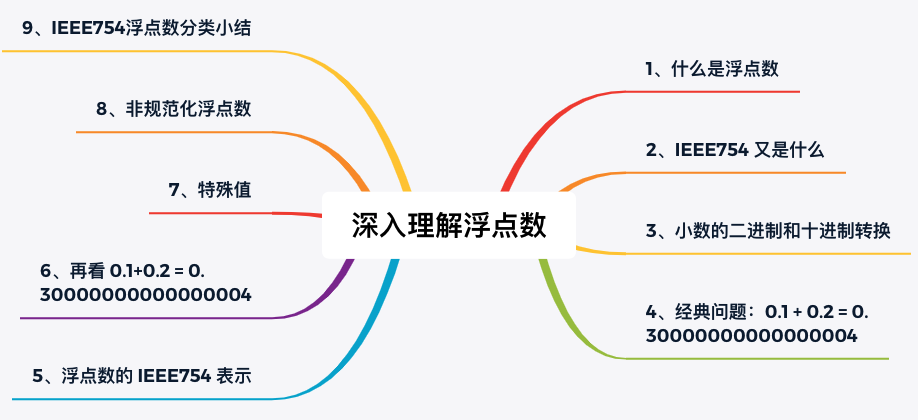## 0、几个问题

• 二进制 0.1，用十进制表示的话是多少？十进制的 0.1，用二进制表示又是多少？
• 为什么 0.1 + 0.2 = 0.30000000000000004？
• 单精度和双精度浮点数的有效小数位分别是多少？
• 单精度浮点数能表示的范围是什么？
• 浮点数为什么会存在 -0？infinity 和 NaN 又是怎么表示的？

## 1、什么是浮点数

The term floating point refers to the fact that a number’s radix point (decimal point, or, more commonly in computers, binary point) can float; that is, it can be placed anywhere relative to the significant digits of the number.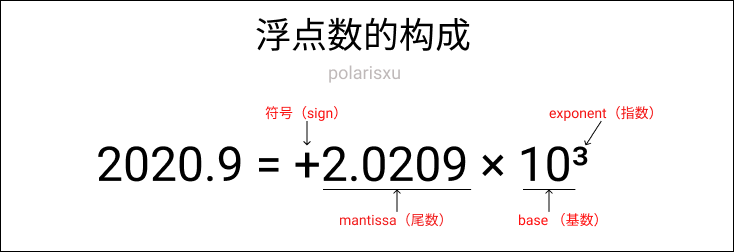## 2、IEEE754 又是什么

The IEEE Standard for Floating-Point Arithmetic (IEEE 754) is a technical standard for floating-point arithmetic established in 1985 by the Institute of Electrical and Electronics Engineers (IEEE). The standard addressed many problems found in the diverse floating-point implementations that made them difficult to use reliably and portably . Many hardware floating-point units use the IEEE 754 standard.

## 3、小数的二进制和十进制转换

### 二进制转十进制

(0.101)₂ = 1×2⁻¹ + 0×2⁻² + 0×2⁻³ = (0.625)₁₀

### 十进制转二进制

``````11/2=5 … 余1
5/2=2  … 余1
2/2=1  … 余0
1/2=0  … 余1
``````

``````0.625*2=1.25 … 取整数部分1
0.25*2=0.5 	 … 取整数部分0
0.5*2=1			 … 取整数部分1
``````

## 4、经典问题：0.1 + 0.2 = 0.30000000000000004

``````#include <stdio.h>

int main(int argc, char** argv) {
printf("%.17f\n", .1 + .2);
return 0;
}
``````

Go 语言：

``````package main

import (
"fmt"
)

func main() {
var a, b float64 = 0.1, 0.2
fmt.Println(a + b)
}
``````

## 5、浮点数的 IEEE754 表示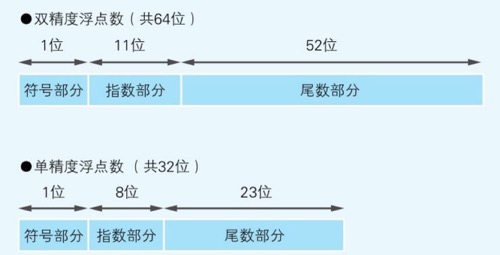## 6、程序确认下 IEEE754 的如上规定

### 一张图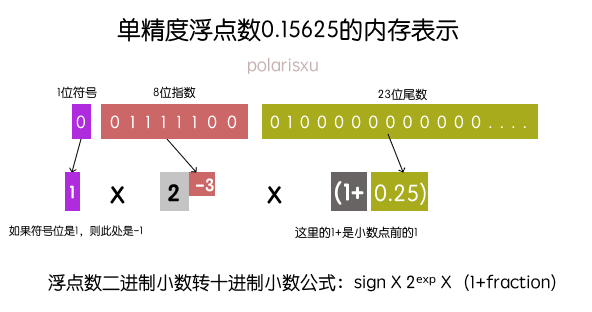### 程序确认单精度浮点数的内存表示

``````package main

import (
"fmt"
"math"
)

func main() {
var f float32 = 0.15625
outputFEEE754(f)
}

func outputFEEE754(f float32) {
// 将该浮点数内存布局当做 uint32 看待（因为都占用 4 字节）
// 这里实际上是做强制转换，内部实现是：return *(*uint32)(unsafe.Pointer(&f))
buf := math.Float32bits(f)

// 加上两处 -，结果一共 34 byte
var result byte

// 从低字节开始
for i := 33; i >= 0; i-- {
if i == 1 || i == 10 {
result[i] = '-'
} else {
if buf%2 == 1 {
result[i] = '1'
} else {
result[i] = '0'
}
buf /= 2
}
}

fmt.Printf("%s\n", result)
}

// output: 0-01111100-01000000000000000000000
``````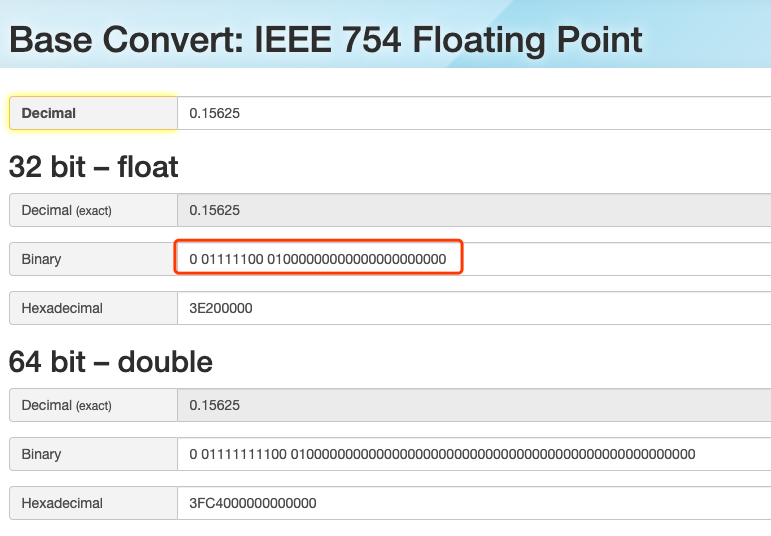## 6、再看 0.1+0.2 = 0.30000000000000004

### 出错的原因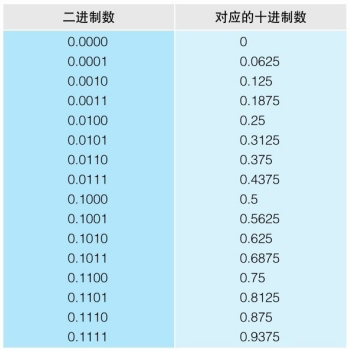### 0.1 和 0.2 是如何表示的？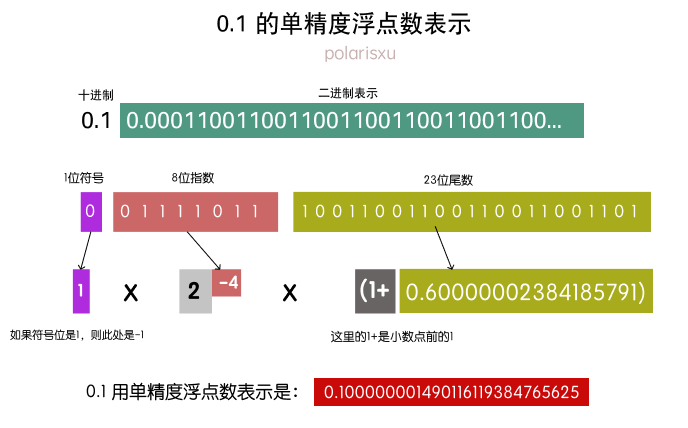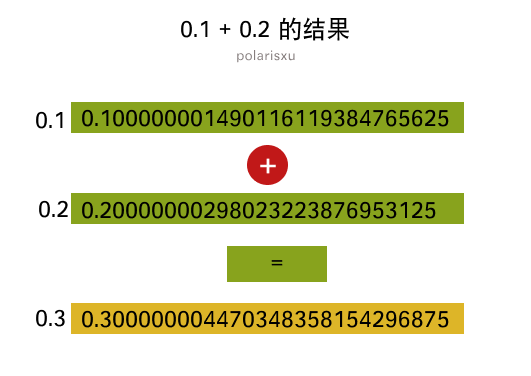## 7、特殊值

### 单精度浮点数的最大值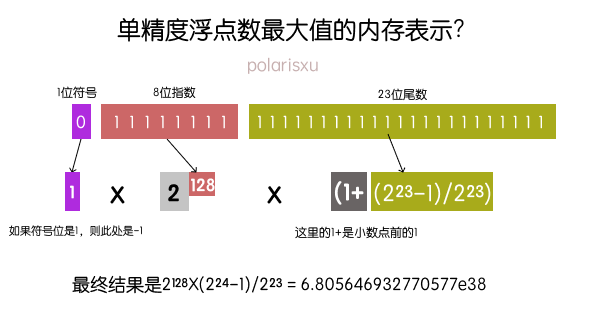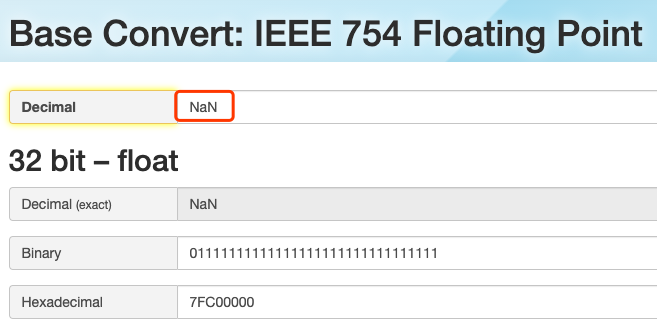### 特殊值 NaN

NaN 是 not-a-number 的缩写，即不是一个数。为什么需要它？例如，当对 -1 进行开根号时，浮点数不知道如何进行计算，就会使用 NaN，表示不是一个数。

NaN 的具体内存表示是：指数位全是 1，尾数位不全是 0。

### 小结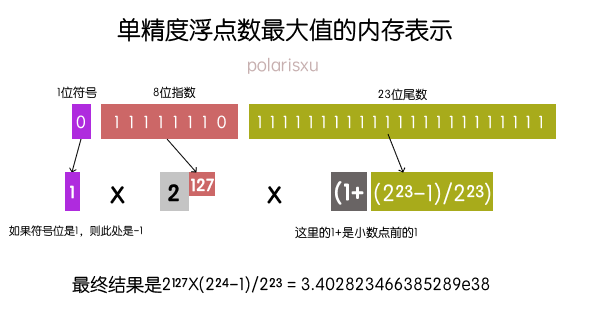## 8、非规范化浮点数## 9、IEEE754 浮点数分类小结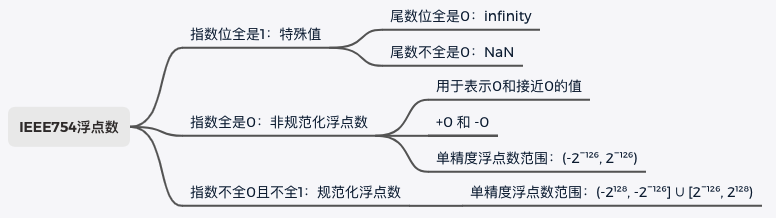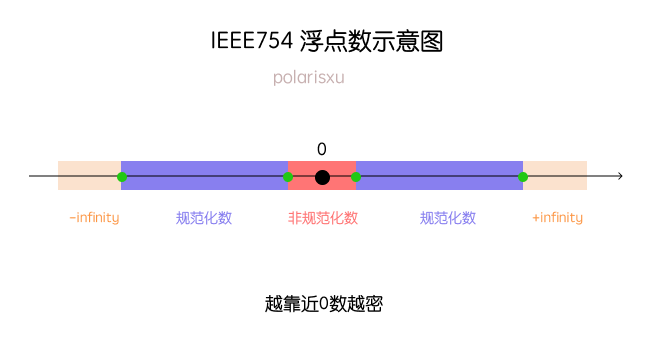## 10、总结

《深入理解计算机系统》这本书在讲解浮点数时说：许多程序员认为浮点数没意思，往坏了说，深奥难懂。经过本文的四千多字图文并茂的方式讲解，如果你认真看完了，我相信你一定掌握了浮点数。

## 参考资料或相关链接5351 字

2020-09-29 18:12 +0800